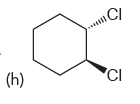Problem:  Identify whether each of the following compounds is chiral or achiral:

FREE Expert Solution
84% (306 ratings)
Problem Details

Identify whether each of the following compounds is chiral or achiral:What scientific concept do you need to know in order to solve this problem?

Our tutors have indicated that to solve this problem you will need to apply the Test 3: Disubstituted Cycloalkanes concept. You can view video lessons to learn Test 3: Disubstituted Cycloalkanes Or if you need more Test 3: Disubstituted Cycloalkanes practice, you can also practice Test 3: Disubstituted Cycloalkanes practice problems .

What is the difficulty of this problem?

Our tutors rated the difficulty of  Identify whether each of the following compounds is chiral ... as low difficulty.

How long does this problem take to solve?

Our expert Organic tutor, Jonathan took half a minute to solve this problem. You can follow their steps in the video explanation above.

What textbook is this problem found in?

Our data indicates that this problem or a close variation was asked in . You can also practice practice problems .